# (1 point) Consider the Initial Value Problem -5 dx dt X x(0) (a) Find the eigenvalues...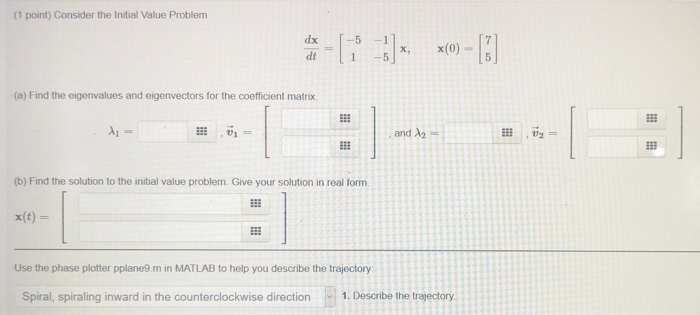(1 point) Consider the Initial Value Problem -5 dx dt X x(0) (a) Find the eigenvalues and eigenvectors for the coefficient matrix A = and 2 -- 1 333 (b) Find the solution to the initial value problem. Give your solution in real form Use the phase plotter pplane9.m in MATLAB to help you describe the trajectory Spiral, spiraling inward in the counterclockwise direction 1. Describe the trajectory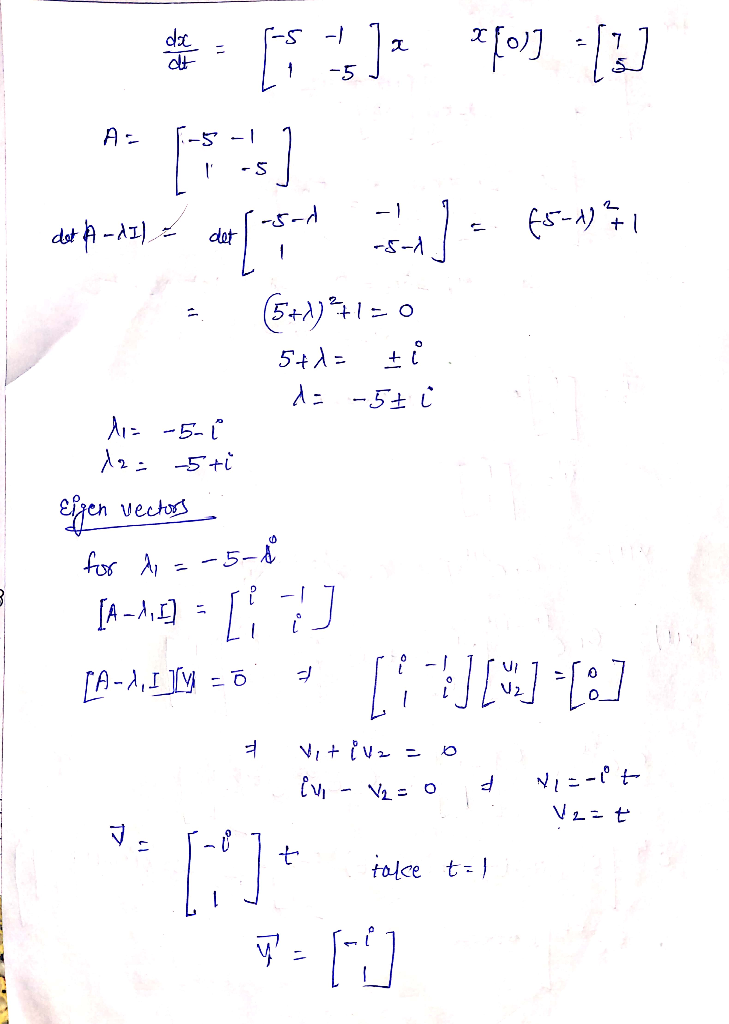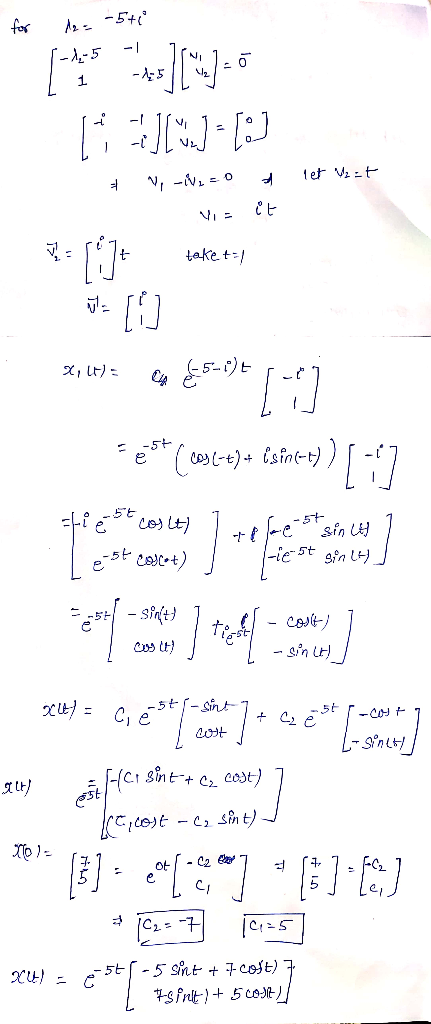have a good day sir please like.it if any doubt please ask in comment thanks alot

#### Earn Coin

Coins can be redeemed for fabulous gifts.

Similar Homework Help Questions
• ### (1 point) Consider the initial value problem (a) Find the eigenvalues and eigenvectors for the coefficient...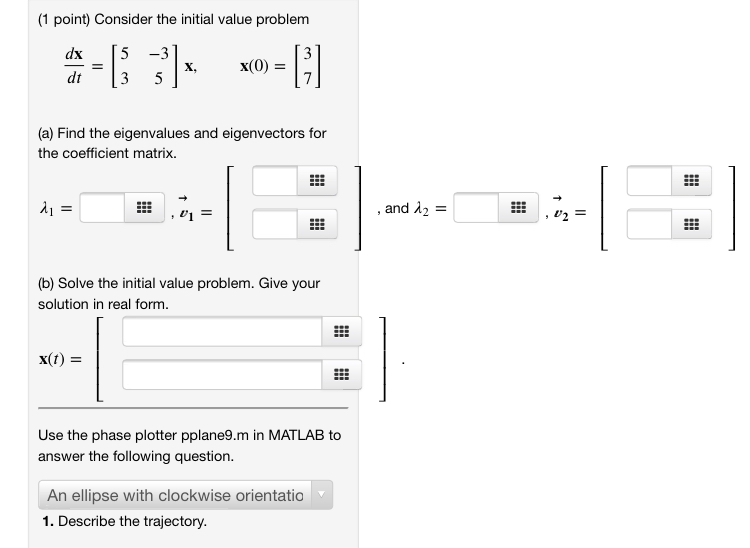(1 point) Consider the initial value problem (a) Find the eigenvalues and eigenvectors for the coefficient matrix. di = , and 12 = (b) Solve the initial value problem. Give your solution in real form. X(t) = Use the phase plotter pplane9.m in MATLAB to answer the following question. An ellipse with clockwise orientatio 1. Describe the trajectory.

• ### (1 point) Consider the initial value problem (a) Find the eigenvalues and eigenvectors for the coefficient...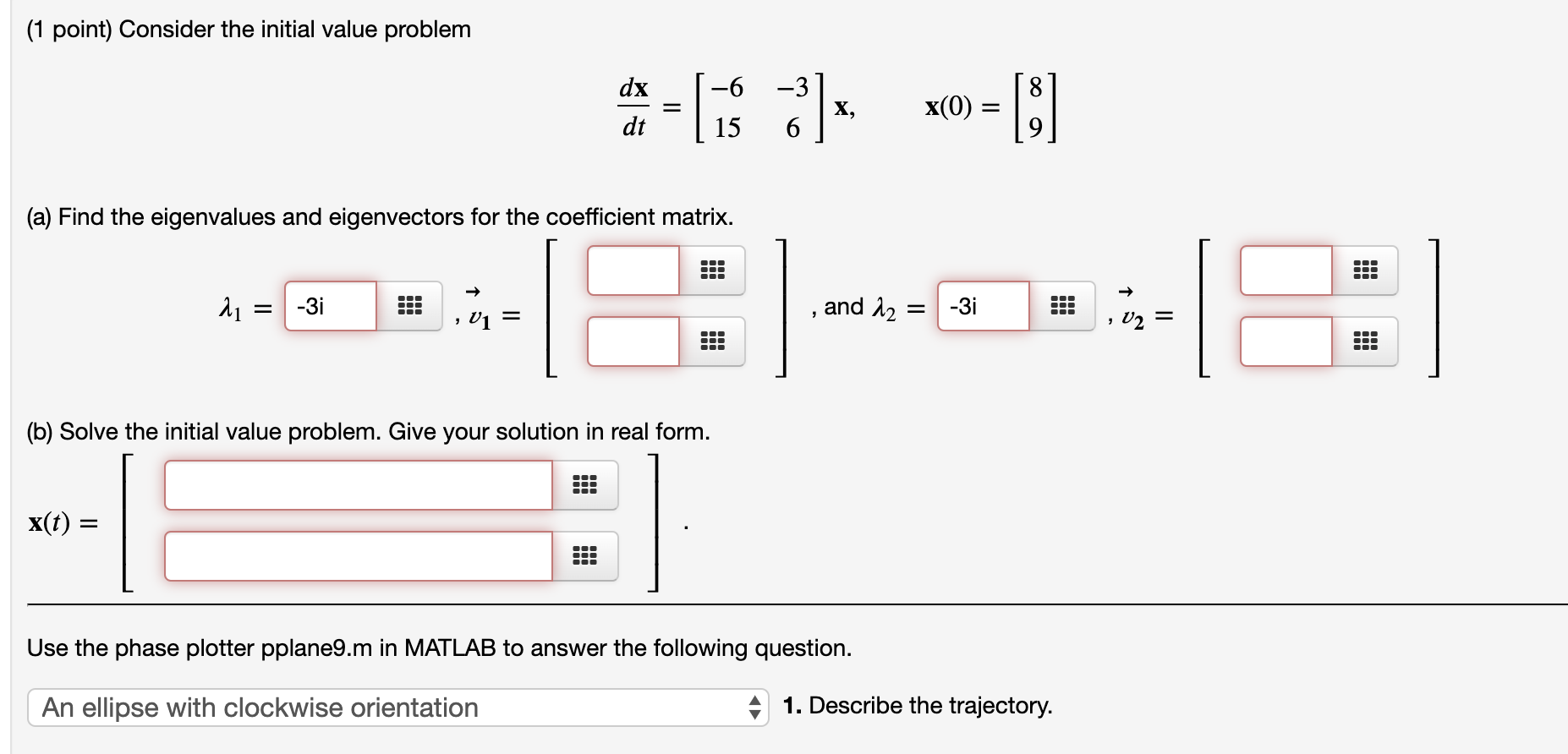(1 point) Consider the initial value problem (a) Find the eigenvalues and eigenvectors for the coefficient matrix. 11 = -3i .. , , and 12 = -3i , 01 (b) Solve the initial value problem. Give your solution in real form. X(t) = Use the phase plotter pplane9.m in MATLAB to answer the following question. An ellipse with clockwise orientation 41. Describe the trajectory.

• ### 7.6(3) (1 point) Consider the Initial Value Problem -L* 4)*, x0=[!] (a) Find the eigenvalues and...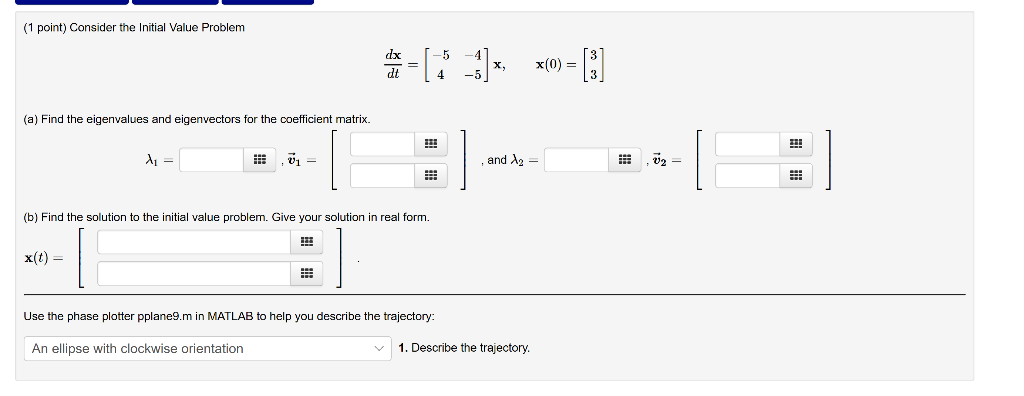7.6(3) (1 point) Consider the Initial Value Problem -L* 4)*, x0=[!] (a) Find the eigenvalues and eigenvectors for the coefficient matrix. 1 ,01 = , and 12 = (b) Find the solution to the initial value problem. Give your solution in real form. x(t) = Use the phase plotter pplane9.m in MATLAB to help you describe the trajectory An ellipse with clockwise orientation 1. Describe the trajectory.

• ### (1 point) Consider the Initial Value Problem 7-177] - (a) Find the eigenvalues and eigenvectors for...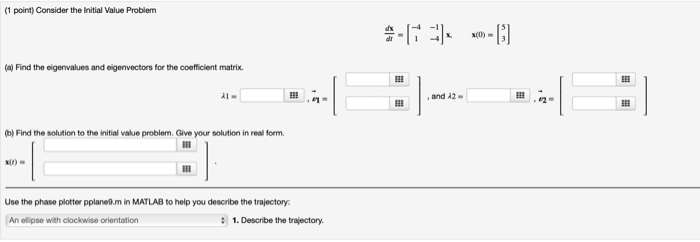(1 point) Consider the Initial Value Problem 7-177] - (a) Find the eigenvalues and eigenvectors for the coefficient matrix. Find the solution to the initial value problem. Give your solution in real form. Use the phase plotter pplanom in MATLAB to help you describe the trajectory An ellipse with clockwise orientation • 1. Describe the trajectory.

• ### Section 7.6 Complex Eigenvalues: Problem 5 Previous Problem Problem List Next Problem (1 point) Consider the...Section 7.6 Complex Eigenvalues: Problem 5 Previous Problem Problem List Next Problem (1 point) Consider the initial value problem date [10 ] x x(0) =  (a) Find the eigenvalues and eigenvectors for the coefficient matrix. X = * , ū = (b) Solve the initial value problem. Give your solution in real form. x(t) = Use the phase plotter pplane9.m in MATLAB to answer the following question An ellipse with clockwise orientation 1. Describe the trajectory

• ### (1 point) Consider the Initial Value Problem: = 's = -9x1 + 3x2 -3021 + 9x2...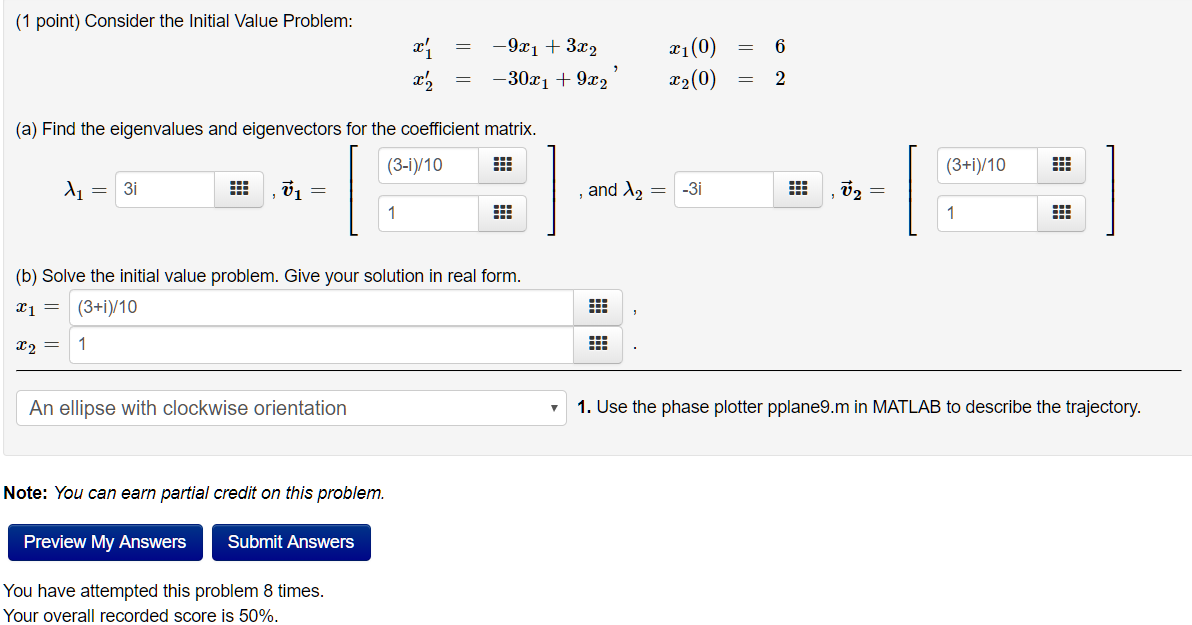(1 point) Consider the Initial Value Problem: = 's = -9x1 + 3x2 -3021 + 9x2 xi(0) = 6 22(0) = 2 (a) Find the eigenvalues and eigenvectors for the coefficient matrix. (3-1)/10 (3+1)/10 l1 = 3i , = , and 12 = -3i , ū2 = | (b) Solve the initial value problem. Give your solution in real form. x1 = (3+1)/10 x2 = 1 An ellipse with clockwise orientation 1. Use the phase plotter pplane9.m in MATLAB to...

• ### 3 of the questions remain unanswered. (1 point) Consider the Initial Value Problem: * = -...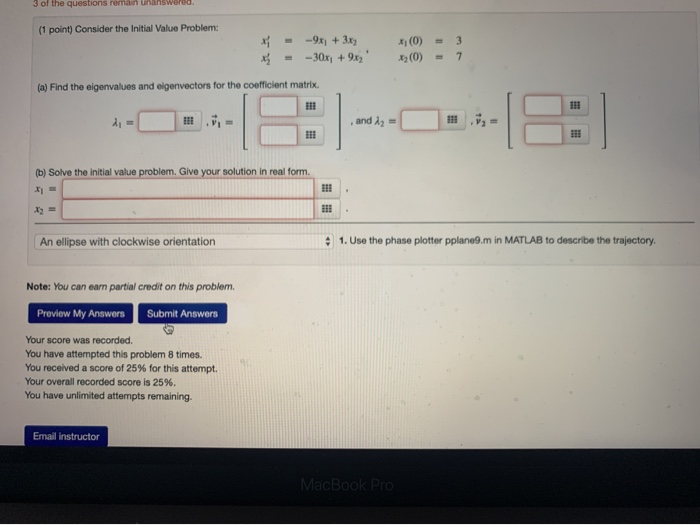3 of the questions remain unanswered. (1 point) Consider the Initial Value Problem: * = - -9x + 3x --30x + 9x2 (0) (0) = - 3 7 (a) Find the eigenvalues and olgenvectors for the coefficient matrix. (b) Solve the initial value problem. Give your solution in real form. An ellipse with clockwise orientation 1. Use the phase plotter pplane9.m in MATLAB to describe the trajectory. Note: You can earn partial credit on this problem. Preview My Answers Submit...

• ### (1 point) Solve the initial value problem dx -H x(0) х, dt Give your solution in...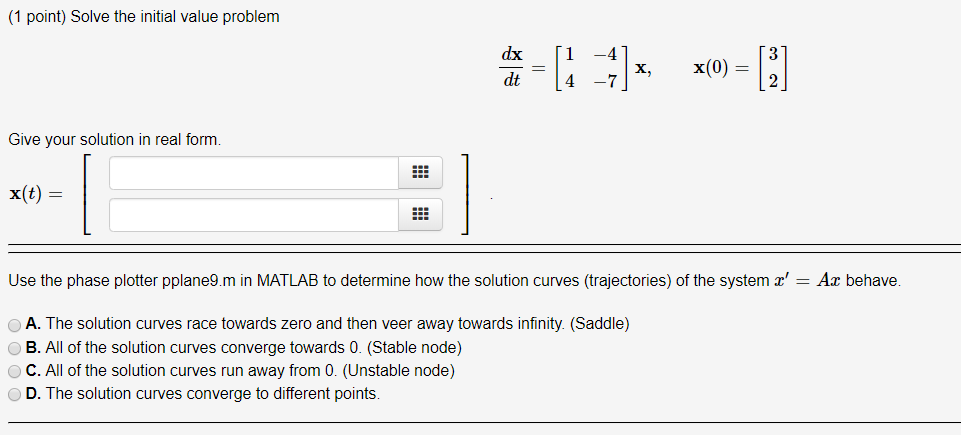(1 point) Solve the initial value problem dx -H x(0) х, dt Give your solution in real form. x(t) Use the phase plotter pplane9.m in MATLAB to determine how the solution curves (trajectories) of the system x' = Ax behave. A. The solution curves race towards zero and then veer away towards infinity. (Saddle) B. All of the solution curves converge towards 0. (Stable node) C. All of the solution curves run away from 0. (Unstable node) D. The solution...

• ### (1 point) Consider the Initial Value Problem xi(0) 6 = 10xi-4x2 (a) Find the eigenvalues and eige...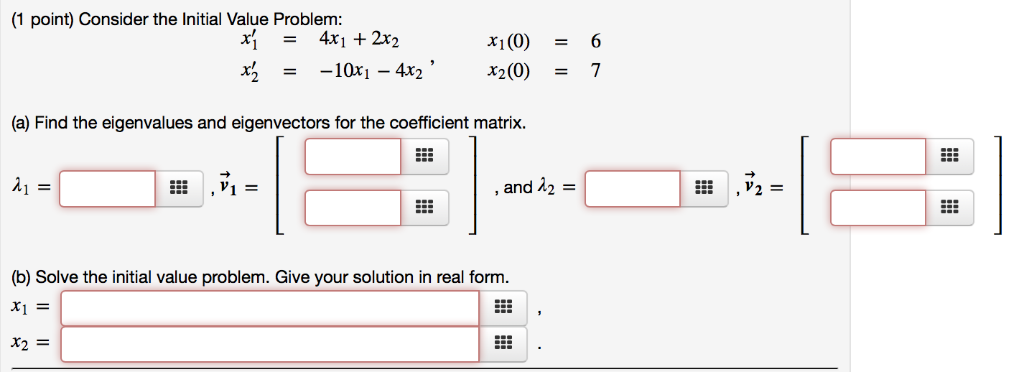(1 point) Consider the Initial Value Problem xi(0) 6 = 10xi-4x2 (a) Find the eigenvalues and eigenvectors for the coefficient matrix. ,V2- and 12 (b) Solve the initial value problem. Give your solution in real form x1F X2= (1 point) Consider the Initial Value Problem xi(0) 6 = 10xi-4x2 (a) Find the eigenvalues and eigenvectors for the coefficient matrix. ,V2- and 12 (b) Solve the initial value problem. Give your solution in real form x1F X2=

• ### (1 point) Solve the initial value problem dx 1.5 2. -1.5 1,5) X, x(0) = (-3)...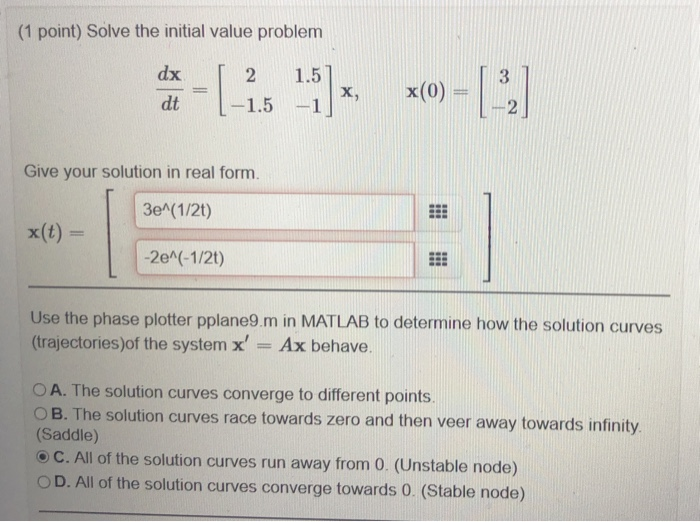(1 point) Solve the initial value problem dx 1.5 2. -1.5 1,5) X, x(0) = (-3) dt -1 Give your solution in real form. 3e^(1/2) x(t) = -2e^(-1/2t) Use the phase plotter pplane9.m in MATLAB to determine how the solution curves (trajectories)of the system x' Ax behave. O A. The solution curves converge to different points. OB. The solution curves race towards zero and then veer away towards infinity (Saddle) C. All of the solution curves run away from 0....